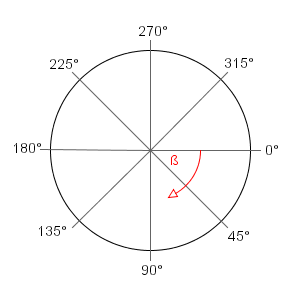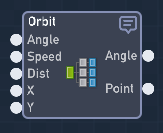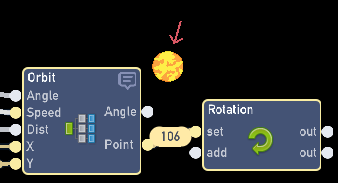# How does the orbit example work?

I’m trying to make an object point toward the mouse, and the axis being this round object. I relize I know almost nothing about circles.

2 Likes

I’m guessing you mean this one?
Flowlab Game Creator - Orbit Example 3

The example simplifies most of it, just the basic understanding of circles would help. It’s also all explained in the code for the Orbit to work. Let me first explain the basics then I can show how you can use that in the code.

## Basics of a circle: Degrees

There are 360 degrees around a circle:(use this graph for video games, the normal graph for school rotates to the left)

Explaining anymore will be confusing, but all you need to know is where each degree is on the circle.
Right = 0, Down = 90, Left = 180, Up = 270

## The Orbit Bundle:• The Angle is where the orbit will start.
Use the Degree to start in that direction. (Right = 0, Down = 90, Left = 180, Up = 270)

• Speed is how fast the orbit is going.
Just play around with it, Speeds around 100 is usually slow.

• Dist is the Distance away from the center of the circle or orbit.
This is in pixels and best to around with it. 100 pixels is usually short.

• X and Y is the center of the orbit / circle.
This is where it will be orbiting around. Using extractors for “Other” objects is best.

Oh yeah, and point is pointing at the center of the orbit / circle, like this:5 Likes

Yes, I understand that, but I don’t understand what equations you used to cauculate what X Y position it should be in. What’s a radian?

1 Like

Radian is just a headache that’ll you’ll learn at the end of your high school and college…

You dont need to look into the code, just copy and import that orbit bundle into your game.

Use the highlight tool, select the bundle and press copy.

Go to your game click the editor and select import from the menu wheel. When the textbox appears, paste the code in there (CTRL + V).

2 Likes

Radians are just a different unit for measuring angles (instead of degrees). It makes the math a little simpler in a lot of cases, so it’s pretty common for doing calculations on them.

2 Likes

I know I know, I just wanted to know how the math worked, but okay. It seemed like fractions and multiplaction at first, but then there’s antan.pi or whatever.Also, thank you, grazer.

1 Like

If you really want to go into the mathmatics @Flying_Fahita, then lets buckle up because I’m going to explain what all the expressions are used for in my Orbit example! I’m going to go in detail, double-check my math, and learn a few things myself because I got some help with this system… so expect changes in the code too.

# Math.atan2(A,B)

What you saw was atan2 was just used for the AngleTo bundle, which is also another example I have that gets the angle between 2 objects. (Angle-To Example). What atan2 does is that it gives the angle compare to the origin.

Like what is the angle (X,Y) from (0,0)?
Example2: atan2 (5,5) = 0.785398 (or 45 degrees)

The expression in the AngleTo bundle is this:
90-(Math.atan2(A,B)/Math.PI) * 180

where
A = Target X - This X
B = Target Y - This Y

# Orbit Bundle:

### Degree to Radian: (This is now updated into the example)

If you input degree’s, it gets changed into radian by using (Degree × π/180). As well in the expression, you can see I multiplied by 1000 so you can have more speed options. For example 0 - 1000 is a good range of speed, but you can also go over 1000. And another note, since Flowlab now allows decimal numbers, I may lower the range to 100 instead to have similar speeds to velocity (in the future).

### Speed:

The speed is the Radian (angle) + speed in which outputs a NEW angle. The expression is looped where the speed is continuously adding speed to the new angle.

### X and Y Positions:

After Speed gives us a new angle, we convert that angle to an X position by using cos(new angle / 1000). To get the Y position, we want to use sine instead of cos. I divide by 1000 because the angle I used to change degrees to radians is being multiplied by 1000.

### Distance:

After cos and sine, we multiply how many pixels away from the center.
Think of it like the Angle * the Distance.

### Center the X and Y Positions:

After we added our distance into the equation, we just need to update to the center of where we want to orbit! This is Simple as Result X + Target X or Result Y + Target Y.

Just make the Speed add a new angle every frame and we have an Orbit!
Angle + Speed = (Position x Distance) + Center Position

And that’s it, the Orbit is basically just updating the position around the object. This also has many more uses other than to make an Orbit, but I made this bundle so its easy for anyone to get a fixed position around an object. I also have example for Cos/Sine, Angle-To, Custom Ease, Bundle Library, and many many more on my profile JR 01.

5 Likes2 Likes

I simplified it as much as I can but still haven’t explained some things or why I did others. If anyone has any specific questions, I’ll go over about it.

1 Like

I was (mostly) kidding. I used to know how to math, even advanced math. But it’s one of those things, if you don’t use it for 20 years, you start to forget. Flowlab has been good for me in that it’s forcing me to use old skills I once had that have been gathering dust. I can probably relearn most of my maths on my own, now that I finally have a reason to.3 Likes

Heh yeah, I’ve more so started teaching myself more since this year started. Studying my custom ease bundle, Cos/Sine and some things I’ve seen on Twitter. Heck, I just edited/fixed an expression in Orbit example because of this discussion.

Math has always been my favorite subject, but I kinda skipped HS precal using Ti-84 and failed college calculus before I changed majors. Studying by using Flowlab has really helped me understand the unit circle.

2 Likes

I did okay in math, and always understood its importance and value, but rarely found any “fun” applications for it. I think if I had access to something like Flowlab when I was in school, I probably would have paid much more attention in math class.

2 Likes

Quick question, @TGW or @JR_01. How do you delete or edit a behavior bundle that is already “behavior menu”? Is it possible? I hope it is… I have a few edits to make.

2 Likes

Hold down on a bundle with left click and hover your mouse over delete, then let go. You can’t delete default bundles.

I’d recommend using a bundle, editing it and deleting the old one, then adding it to the behavior bundle menu afterwards.

@Flying_Fahita

3 Likes